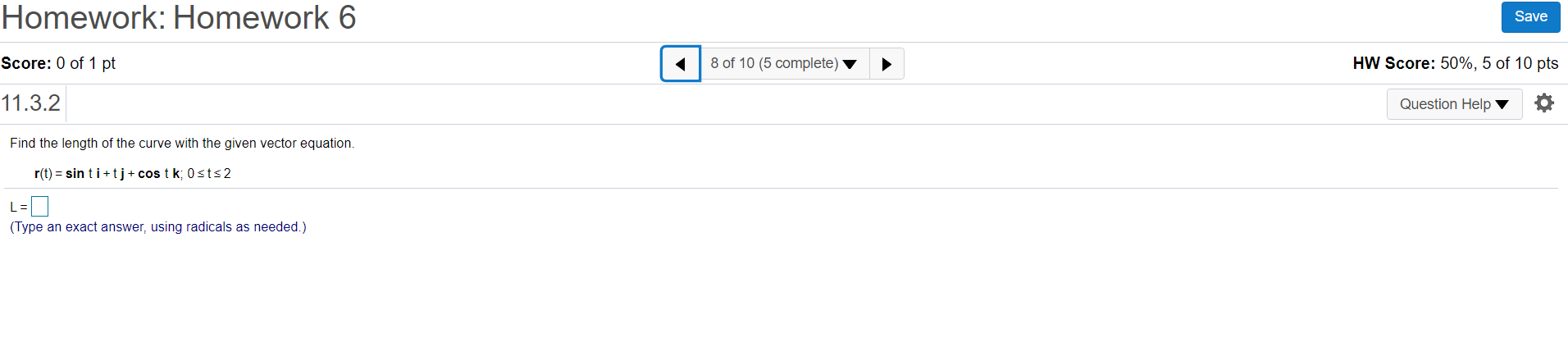### Create an Account

Home / Questions / Homework: Homework 6 Save Score: 0 of 1 pt 8 of 10 (5 complete) HW Score: 50%, 5 of 10 pts...

# Homework: Homework 6 Save Score: 0 of 1 pt 8 of 10 (5 complete) HW Score: 50%, 5 of 10 pts 11.3.2 Question Help Find the length of the curve with the given vector equation. r(t) = sin ti+t] + cos tk;0

Homework: Homework 6 Save Score: 0 of 1 pt 8 of 10 (5 complete) HW Score: 50%, 5 of 10 pts 11.3.2 Question Help Find the length of the curve with the given vector equation. r(t) = sin ti+t] + cos tk;0sts2 L=0 (Type an exact answer, using radicals as needed.)Apr 28 2021 View more View LessSubscribe To Get Solution